# Tetrakis hexahedron

Tetrakis hexahedron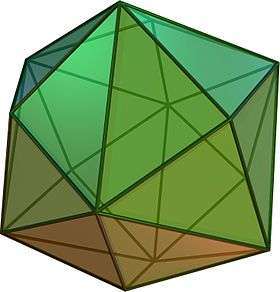TypeCatalan solid
Coxeter diagramConway notationkC
Face typeV4.6.6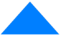isosceles triangle
Faces24
Edges36
Vertices14
Vertices by type6{4}+8{6}
Symmetry groupOh, B3, [4,3], (*432)
Rotation groupO, [4,3]+, (432)
Dihedral angle143°07′48″
arccos(−4/5)
Propertiesconvex, face-transitive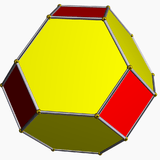Truncated octahedron
(dual polyhedron)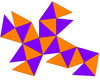Net

In geometry, a tetrakis hexahedron (also known as a tetrahexahedron, hextetrahedron and kiscube) is a Catalan solid. Its dual is the truncated octahedron, an Archimedean solid.

It also can be called a disdyakis hexahedron as the dual of an omnitruncated tetrahedron.

## Orthogonal projections

The tetrakis hexahedron, dual of the truncated octahedron has 3 symmetry positions, two located on vertices and one mid-edge.

Projectivesymmetry Tetrakishexahedron Truncatedoctahedron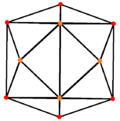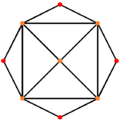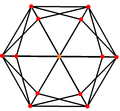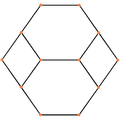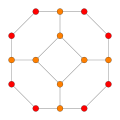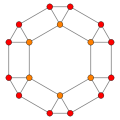## Uses

Naturally occurring (crystal) formations of tetrahexahedra are observed in copper and fluorite systems.

Polyhedral dice shaped like the tetrakis hexahedron are occasionally used by gamers.

A 24-cell viewed under a vertex-first perspective projection has a surface topology of a tetrakis hexahedron and the geometric proportions of the rhombic dodecahedron, with the rhombic faces divided into two triangles.

The tetrakis hexahedron appears as one of the simplest examples in building theory. Consider the Riemannian symmetric space associated to the group SL4(R). Its Tits boundary has the structure of a spherical building whose apartments are 2-dimensional spheres. The partition of this sphere into spherical simplices (chambers) can be obtained by taking the radial projection of a tetrakis hexahedron.

## Symmetry

With Td, [3,3] (*332) tetrahedral symmetry, the triangular faces represent the 24 fundamental domains of tetrahedral symmetry. This polyhedron can be constructed from 6 great circles on a sphere. It can also be seen by a cube with its square faces triangulated by their vertices and face centers and a tetrahedron with its faces divided by vertices, mid-edges, and a central point.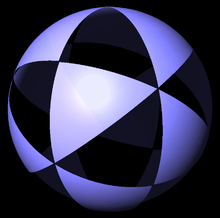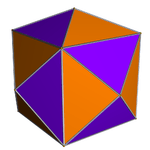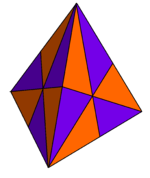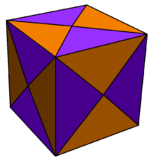The edges of the tetrakis hexahedron form 6 circles (or centrally radial lines) in the plane. Each of these 6 circles represent a mirror line in tetrahedral symmetry. The 6 circles can be grouped into 3 sets of 2 pairs of orthogonal circles. These edges can also be seen as a compound of 3 orthogonal square hosohedrons.

stereographic projection Perspective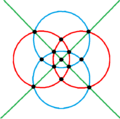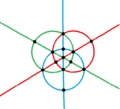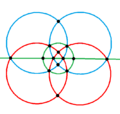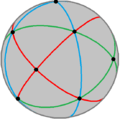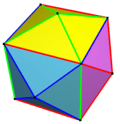Skew

## Dimensions

If we denote the edge length of the base cube by a, the height of each pyramid summit above the cube is a/4. The inclination of each triangular face of the pyramid versus the cube face is arctan(1/2), approximately 26.565° (sequence A073000 in the OEIS). One edge of the isosceles triangles has length a, the other two have length 3a/4, which follows by applying the Pythagorean theorem to height and base length. This yields an altitude of 5a/4 in the triangle (). Its area is 5a/8, and the internal angles are arccos(2/3) (approximately 48.1897°) and the complementary 180°  2 arccos(2/3) (approximately 83.6206°).

The volume of the pyramid is a3/12; so the total volume of the six pyramids and the cube in the hexahedron is 3a3/2.

## Kleetope

It can be seen as a cube with square pyramids covering each square face; that is, it is the Kleetope of the cube.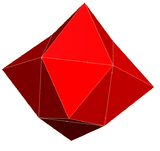## Cubic pyramid

It is very similar to the net for a cubic pyramid, as the net for a square based is a square with triangles attached to each edge, the net for a cubic pyramid is a cube with square pyramids attached to each face.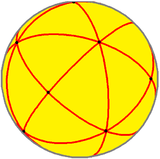Spherical tetrakis hexahedron

It is a polyhedra in a sequence defined by the face configuration V4.6.2n. This group is special for having all even number of edges per vertex and form bisecting planes through the polyhedra and infinite lines in the plane, and continuing into the hyperbolic plane for any n  7.

With an even number of faces at every vertex, these polyhedra and tilings can be shown by alternating two colors so all adjacent faces have different colors.

Each face on these domains also corresponds to the fundamental domain of a symmetry group with order 2,3,n mirrors at each triangle face vertex.